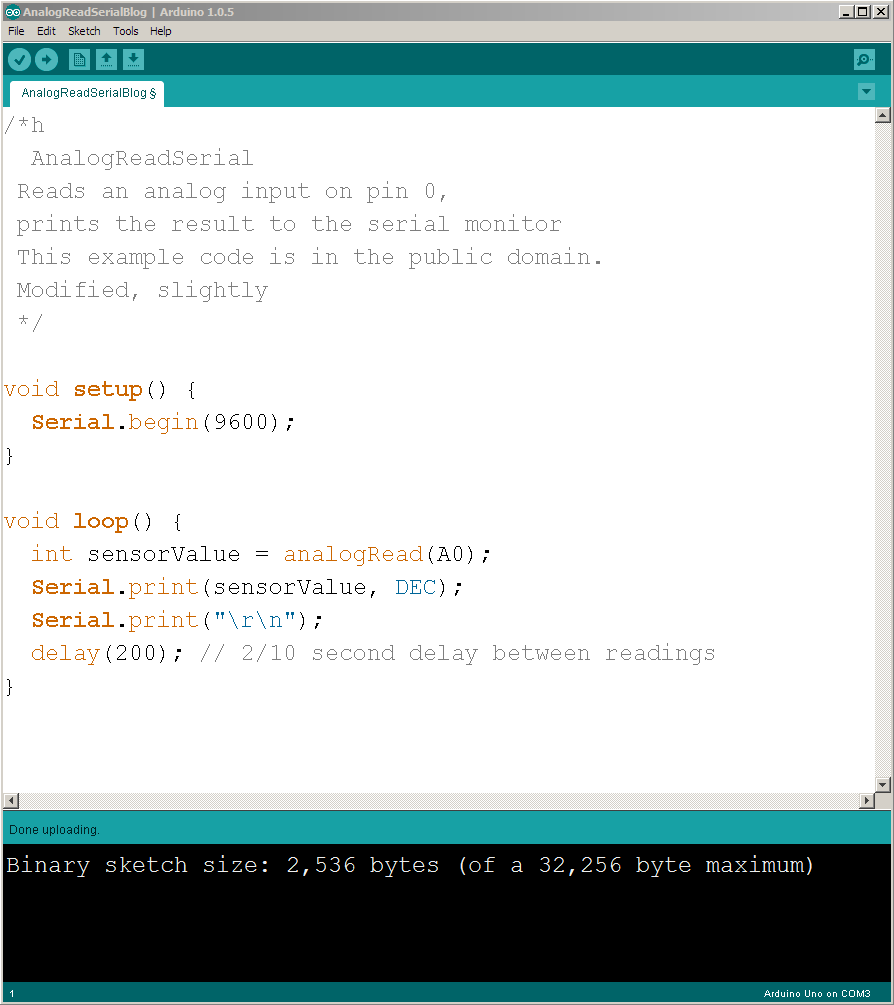# Real Time Data Capture – Using Arduino and JMP

This is the Video Tutorial based off of JMP’s  Craige_Hales  Blog Post. Thank you Craige.

The Arduino is a small cheap but quality microcomputer.  There are tons of tutorials including a free Arduino For Absolute Beginners Course.

This project and video will connect an Arduino to JMP and do some real-time data logging.

You’ll need the Free Software Arduino IDE download

Here’s an image of the Software (IDE)  with the Sketch (Code/Program).This tiny program does two things: initialize the serial communications when the power is turned on, and then spend the rest of its life reading an analog signal and printing the value to the serial port.

Schematic

The sensor for this project is a CdS photo-resistor.  Here’s a picture of the breadboard circuit.the photo-resistor (round with squiggly line, center rear) and the 43K ohm resistor (yellow-orange-orange-gold) next to it form a voltage divider across the black wire (ground) and red wire (5 volt) power supply.  The white wire on the center tap of the divider goes to A0 on the blue Arduino UNO board.  A0 is the analog input the program above is reading.  When the photo-resistor is dark, its resistance is very high (compared to the 43K ohm resistor, and the white wire’s voltage will be near ground (0 volts).  When brightly lit, the photo-resistor’s resistance is very low and the white wire will be close to 5 volts.

You used the USB cable as a serial port to load the program into the Arduino.  It might be com3 or com4 or maybe another choice.  JMP will be listening on that same USB serial connection.  That’s a cool blue USB cable that might come with the Arduino, depending on the vendor.What makes this tick – JMP Scripting Language JSL

Make a data table with two columns; the time column will be filled in with the number of seconds since the capture began.  The sense column will be filled in with the data from the Arduino.

```dt = New Table( "capture",
Add Rows( 0 ),
New Column( "time", Numeric, Continuous, Format( ":day:hr:m:s", 21, 3 ) ),
New Column( "sense", Numeric( 2 ) ) // the analog sensor is 0..1023
);```

remember the start time

`start = Tick Seconds();`

Open JMP’s Datafeed, set up the parameters, and supply a script to process each line of data from the Arduino. COM3 is probably not what your Arduino is connected to.

```feed = Open Datafeed(
Connect( Port( "com3" ), Baud rate( 9600 ), Parity( even ), DataBits( 7 ) ),
Set Script(
x = feed << getLine;
dt << addrows( 1 );
dt:time = Tick Seconds() - start;
dt:sense = Num( x );
)
);```

Run a control chart (or a graph builder script) to see the data collection in action.

`dt << Control Chart( Sample Size( 1 ), KSigma( 3 ), Chart Col( :sense, Run Chart() ) );`

When we’re done we’ll see the control chart updating as the data arrives; the data values change depending on the light that reaches the sensor.  We’ll also see the axis scrolled to display more of the data and notice a lag in the response time while JMP catches up with the data.  There is no handshaking; it is quite possible for data to be lost.  The 200ms pause in the Arduino code (way above) is designed to give JMP time to process the data and update the display.

Let me know if you have any questions.

Tags:
0 replies